Introduction | Catalog | Tutorial | LaTeX2e Import MetaPost A Very Brief TutorialBy Urs Oswald MetaPost PDF version (340 KB)
 Versions Used MetaPost 0.641 latex2HTML 2002-1 (1.68), May 2002 pdflatex 3.14159-1.00a

Last update: October 8, 2002

 A2B LineSegments Circles CircleParametrization BezierCurves GraphSqrt Paths StringLabels TeXLabels LaTeXLabels Equations Mediation Precedence whatever Directions Times Colors Slanted Scaled xScaled zScaled Transform Functions ForSuffixes Recursiveness RecursivePath ifthenInLabel NewOperators DynamicTeXLabels TextOnCurve

A2B.mp

The diagonal line segment at the right is drawn by the MetaPost command

draw (100, 100)--(350, 275).

The unit of MetaPost is the PostScript point which is 1/72 of an inch. The default line thickness is 0.5 PostScript points, as is seen from A2B.1. This is the file generated as one result of the command

mpost A2B.(On other systems, the command may be mp A2B.) The other resulting file is A2B.log.

TeX notation for a PostScript point is "bp" (big point). TeX allows also "pt", which is slightly less than one "bp":

1pt is 1/72.27 of an inch.

LineSegments.mp

• draw
• drawarrow
• fill
• pickup pencircle
The command pickup pencircle scaled 2 increases line thickness to 2 PostScript points. With pickup pencircle scaled .5, it is set back to normal (default).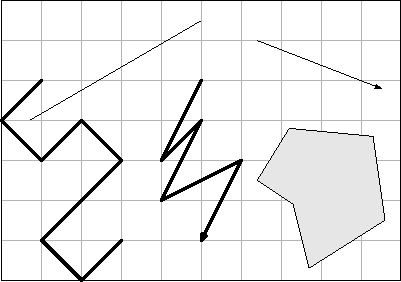Circles.mp

• fullcircle
• halfcircle
• scaled
• xscaled
• yscaled
• rotated
• shifted
In mathematics as in MetaPost, the positive sense of rotation is counterclockwise. Thus the red halfcircle is obtained (from the black one) by a rotation of +90 degrees, whereas the blue halfcircle is obtained by a rotation of -90 degrees.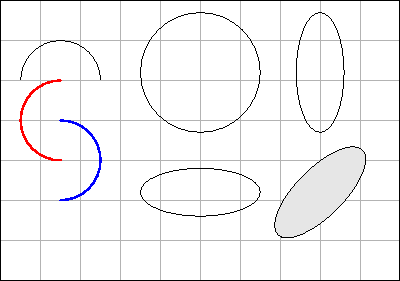CircleParametrization.mp

• point t of p
• subpath (s, t) of p
• cutbefore
The points on a fullcircle have parameter values ranging from 0 to 8. If a path is transformed (such as scaled or rotated), the points keep their parameter values.

The ellipse at the lower left has undergone a rotation of 225 degrees. Therefore the first half lies upside down (bold red color).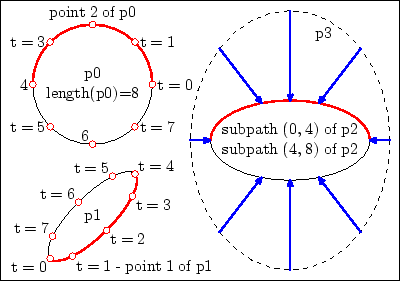The blue arrows in the right half of the figure are directed to the center of the inner ellipse. They are
cutbefore subpath (0, 4) of p2

(bold red) if they start from above the center of p2, and

cutbefore subpath (4, 8) of p2
otherwise.

BezierCurves.mp

• cubic Bezier curve
• control points
Each pair of consecutive points is connected by a cubic Bézier curve which needs, in order to be determined, two intermediate control points in addition to the end points.

In the opposite figure, the additional control points and the connecting line segments are drawn in red color.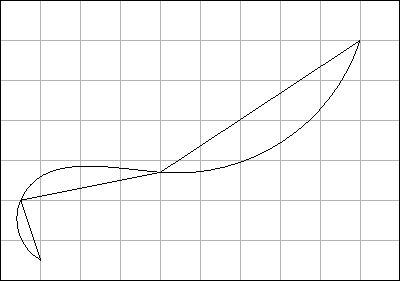MetaPost automatically calculates the control points in such a way that the segments have the same direction at the interior knots.

Cubic Bézier curve (applet)      Mathematics

GraphSqrt.mp

• z points
• direction
Curves can be controlled by directions at the knots.

The black curve has been given the direction {up} at the origin, while the gray curve has been given the same knots, but no direction.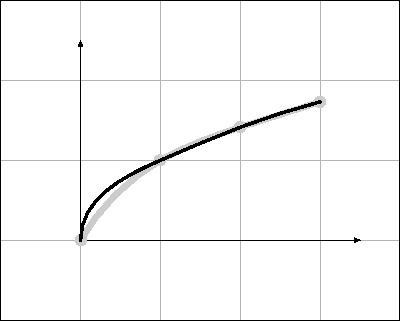Paths.mp

MetaPost paths are objects, as are numerics or strings. MetaPost objects can be combined to form arrays. MetaPost arrays can be addressed like, e.g., Java arrays.

In MetaPost, however, array indexes are not restricted to nonnegative integers (i. e. natural numbers and 0).

In the figure to the right, the rectangles are path objects combined in an array called r[].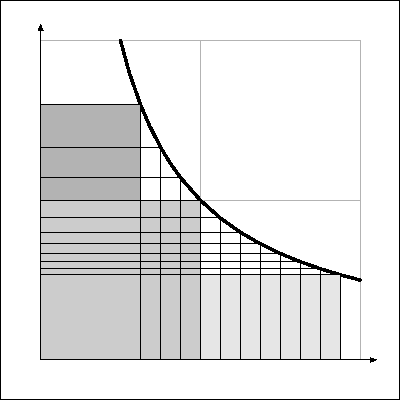StringLabels.mp

• string
• catenation
• label
• label.lft
• label.rt
• label.top
• label.bot
• label.ulft
• ...
• label.lrt
• dotlabel
• decimal
MetaPost strings are objects, as are pairs or paths. string objects (as well as path objects) can be catenated with the operator &

The label commands allow string objects to be written into MetaPost graphics.

The decimal operator generates a string object from a numeric object.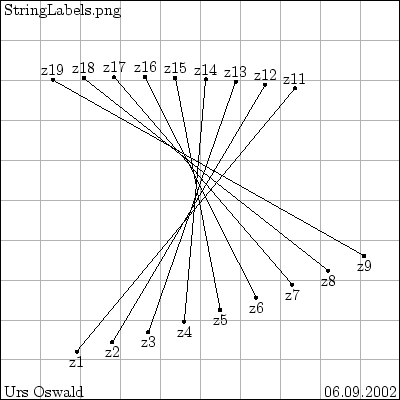TeXLabels.mp

• btex...etex
• verbatimtex...etex
Instead of strings, the label commands accept TeX commands. These have to be enclosed in «btex ... etex».

The result is a MetaPost picture which can, e.g., be transformed like any MetaPost path, pair, or picture (or pen or transform). The labels at the right side of the opposing picture have been rotated by 90 degrees (bottom), and -90 degrees (top).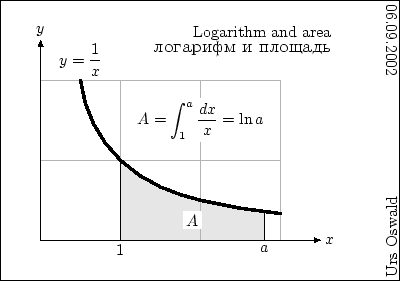The btex...etex commands cannot, alas, be passed to the label commands as MetaPost strings. Background TeX commands like «\font\cyr=wncyr10» can be executed if enclosed in verbatimtex..etex.

LaTeXLabels.mp

• btex...etex
• verbatimtex...etex
• tabular
• NFSS
• \newfont
If you want to use LaTeX, e.g. the New Font Selection System (NFSS), or commands like \begin{tabular} ... \end{tabular} or \newfont{}{}, you have to use the «verbatimtex ... etex» construction.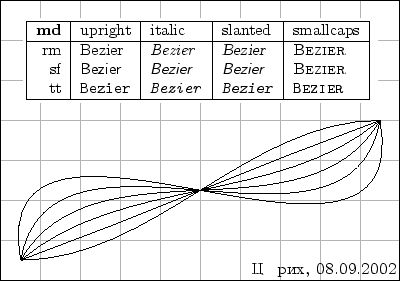The line %&latex (see LaTeXLabels.mp) works in the SuSE-Linux distribution. This line must not have any leading empty spaces.

On other systems, the reference to LaTeX must be made somewhat differently. See, e.g., Die TeXnische Komödie 1/2002, p. 27, where the following possibility is mentioned:
• mp(ost) --tex=latex file name,
On a Unix system, the replacement for the line %&latex may be
• setenv TEX latex file name,
(Alan Hoenig ,TeX UNBOUND).

Comparison of the two images shows that LaTeX2html does not render all the special signs correctly. The two images were both made from one and the same LaTeXLabels.mp. For the picture on top, LaTeX2html (version 2002 - 1) was used. The bottom picture was produced with pdfLaTeX, and the resulting pdf file was converted into a png picture.

By the way, the cyrillic name at the lower right means «Zürich».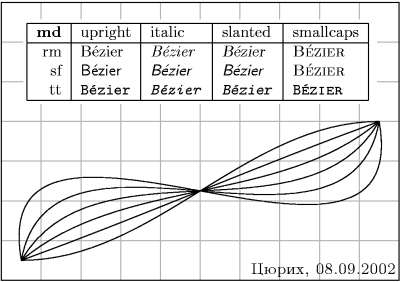Equations.mp

MetaPost is capable of solving systems of linear equations. The equality sign is characteristic for a MetaPost equation, whereas assignments are primarily made by :=. If you demand impossible things like

*c+2b=1;
*2c+4b=1;

MetaPost will reply as follows:

! Inconsistent equation (off by -1),

meaning that 2c+4b has to be equal to 2 if c+2b=1.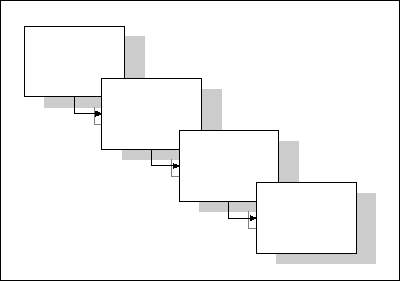Of course, if a has not been defined before, the equation a=5 is completely equivalent to the assignment a:=5.

Equations are very convenient, i.g., to calculate db in the picture to the right. If b0, b1, b2, and B are defined, then db can simply be determined by

b0+B/2+3db+B/2+b1+b2=breite.

MetaPost will solve this equation in order to get the value of db. An analogous equation determines the value of the vertical displacement dh from one window to the next.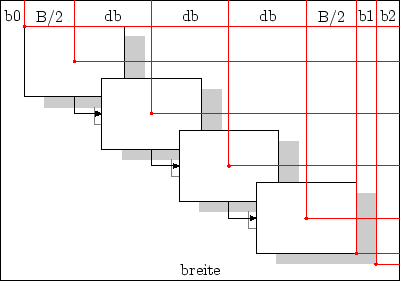Mediation.mp

• r[P, Q]
For given pair objects P, Q, the «mediation expression» r[P, Q] denotes a pair R determined by

R-P = r(Q-P).

The numeric r can have any (MetaPost) real value.

From the above definition of the mediation expression, it follows that

R = (1-r)P + rQ.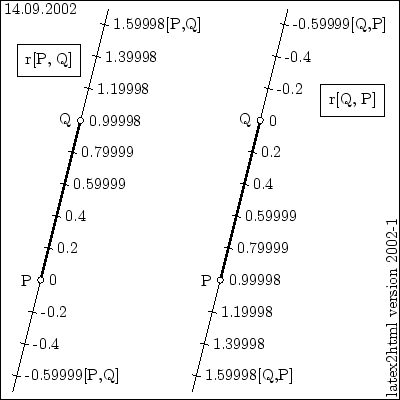The last relation is exactly the one we needed to define Bézier curves! We could define a Bézier curve of arbitrary order recursively by

(1) B0(t, P0) := P0,
(2) Bn(t, P0, ..., Pn) := t[ Bn-1(t, P0, ..., Pn-1),  Bn-1(t, P1, ..., Pn) ]   for n > 0.

In the figure, the mediation values are supposed to be multiples of 1/5. The slight differences are a consequence of the fact that MetaPost internally converts all numeric values to multiples of 1/65536 which is approximately equal to 0.00002.

Precedence.mp

The opposite figure reveals how MetaPost reads mediation expressions.
• 2/5[P0, P1]´  is read as

(2/5)[P0, P1]´.

• -2/5[P0, P1]´  is read as

-((2/5)[P0, P1])´

which is equivalent to

2/5[P0, P1] rotated 180´.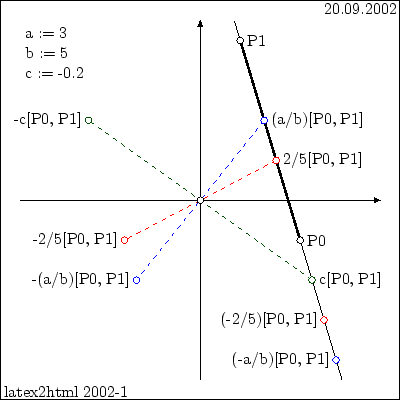MetaPost processes an input stream in two steps:
• It breaks it down into tokens.
• It puts the tokens together to form expressions.
Expressions are classified as to the priority with which they are processed by MetaPost. Expressions of level primary are evaluated first. Multiplicative expressions have level secondary; they are evaluated next. Additive expressions have level tertiary. Everything above tertiary is simply of level expression. Every primary expression is also secondary, etc. (but not vice versa!).

The figure below shows how numeric expressions are classified according to their level.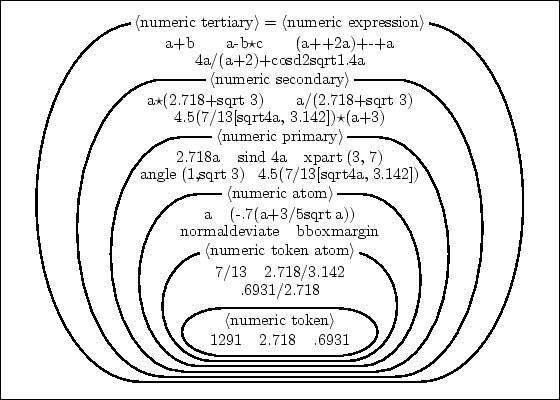whatever.mp

The point of intersection z5 can be obtained with the «intersectionpoint» operator from the paths z1--z3 and z2--z4.

However, as the paths z1--z4 and z2--z3 do not intersect, z7 cannot be obtained in the same way from these two paths. Instead, z7 is obtained by the equation

z7=whatever[z1, z4]
=whatever[z2, z3].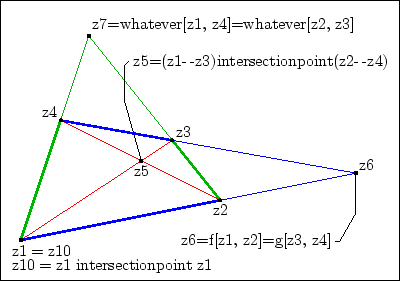The «double equation» z7=whatever[z1, z4]=whatever[z2, z3] could be replaced with the two equations z7=whatever[z1, z4] and z7=whatever[z2, z3].

z6 is obtained by the equation z6=f[z1, z4]=g[z2, z3]. However, while the numeric variables f and g cannot be used in further equations, the whatever can be used over and over again.

By the way: Omitting the parentheses in (z1--z3)intersectionpoint(z2--z4) would cause MetaPost to try to calculate z1--(z3 intersectionpoint z2)--z4, as «intersectionpoint» is a secondary-level operator, while «--» is of expression level, i.e. has minimum precedence. As the points z3 and z2 do not coincide, this attempt would result in an error message saying that the paths z3 and z2 do not intersect. For MetaPost, pairs like z2 and z3 are special paths, namely paths with length zero.

Both whatever and intersectionpoint are examples of macros defined in the plain.mp file; they are not built-in MetaPost commands. This file shows how to define new functions and operators. The binary operator intersectionpoint is defined with the use of the built-in operator intersectiontimes. The ?' in the definition of whatever is just an arbitrary variable name. MetaPost allows variable names like ?', !', or any combination of these two signs.

Directions.mp

• direction
• tension
Have you ever tried to digitize your signature? I gave up after the first initial. Of course, once you are successfull, you copy the result over and over again.

Directions can be indicated, e.g., in the form {2,3} or, equivalently, {(2,3)} - which means the slope of a line segment going 2 units to the right and 3 units up. {up} is just an abbreviation for (0,1).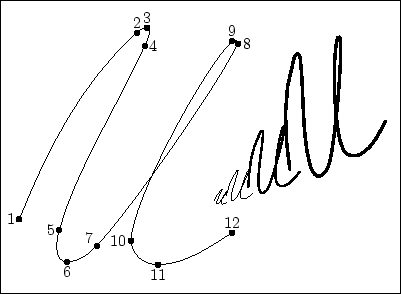Times.mp

In the figure to the right, p0 is defined as

z0..z1..z2..z3.

These knots have integer parameter values i=0, 1, 2, 3. Every point of p0 can be obtained with the command

point t of p0,

and the slope of the tangent with

direction t of p0.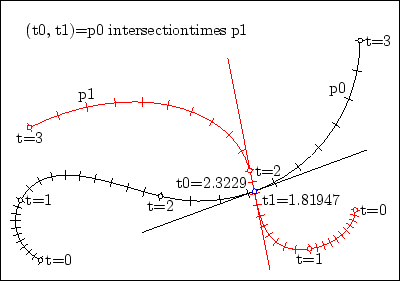Colors.mp

MetaPost objects of type color are triplets of numerics which define colors in the RGB system. The components of a color object col can be obtained with
• redpart col
• greenpart col
• bluepart col
When used to define colors, i.e. with the command withcolor, components have to be in the range of 0 to 1. Apart from this use, they can be any MetaPost objects of type numeric.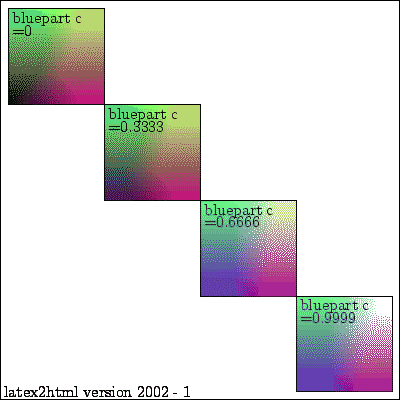Like objects of type pair, objects of type color can be multiplied with, and divided by, objects of type numeric, and any two of them can be added. There lack, however, operators like unitvector or abs.

For some reason, latex2html didn't get the colors quite right. Therefore I used pdflatex and gimp to make a second picture from the same MetaPost source file.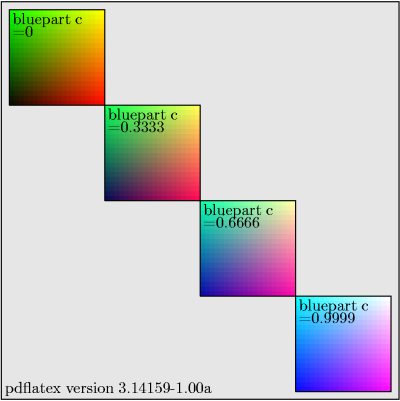Slanted.mp

On any given level, the width of the transformed figure remains unchanged while the x-coordinate is multiplied by the slant factor. The slant factors in this example are 3/7, 6/7, 9/7. On the level y=7, they produce horizontal shifts of 3, 6, and 9, respectively.

The map which causes the slant effect is called a shear (=Scherung in German).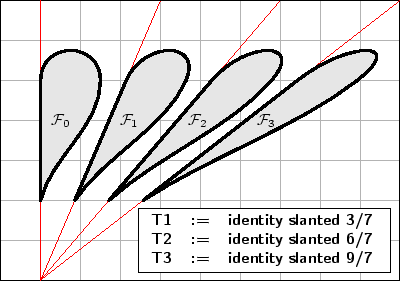Scaled.mp

• btex...etex
• verbatimtex...etex
• subroutine: «draw_point»
A MetaPost transform has 6 components which can be obtained by the operators
• xpart
• ypart
• xxpart
• xypart
• yxpart
• yypart
(See Scaled.mp ).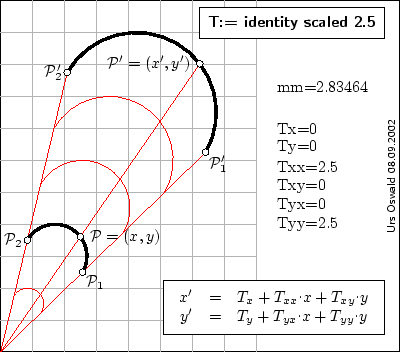The meaning of the 6 components for the calculation of x' and y' from x and y can be seen from the box at the lower right.

For MetaPost, mm is a purely numerical value (the ratio of 1mm to 1bp). So 1 millimeter has 2.83464 PostScript points (bp for TeX).

xScaled.mp

On any given level, the width of the transformed figure is multiplied by 4.

The 6 components of transform T have values

Tx=0
Ty=0
Txx=4
Txy=0
Tyx=0
Tyy=1.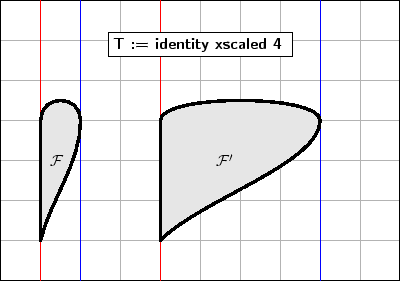zScaled.mp

The command
• zscaled (a,b)
defines a rotation around the origin, followed by a scaling from the origin (or vice versa) with the effect of mapping (0,1) onto (a,b). In the complex plane, this transformation can be achieved by multiplikation with the complex number a + b.i.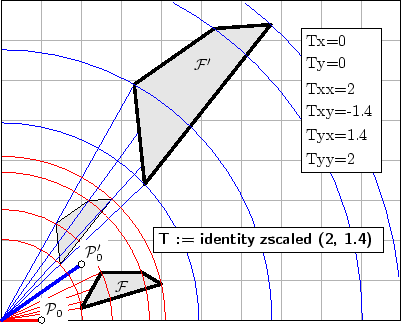As you can see from zScaled.mp, the values of the components of transform T are again obtained by the commands

xpart T, ypart T, xxpart T, xypart T, yxpart T, yypart T.

Transform.mp

MetaPost transforms can be chained together to form new transforms. If t1 and t2 are transforms, then by the command,

t3 := t1 transform t2,

a new transform t3 is defined.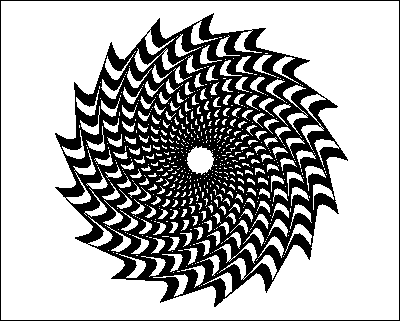The way to get one jagged wheel from the previous one is by the transformation

zscaled P4.

(Notice that the center ist (0,0), and that the point (1,0) is mapped onto the point P4.)

As all picture elements are originally definied in neutral mathematical coordinates (ranging from -1 to 1), they can as well be used for the inserted box, after redefining the transformation t which maps the mathematical coordinates onto the MetaPost picture coordinates.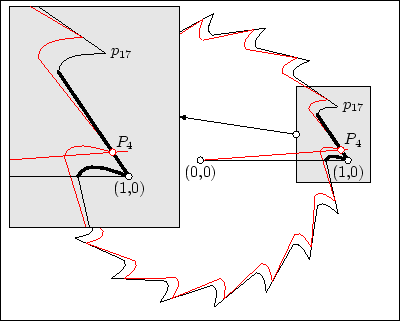Functions.mp

Subroutines written with def...enddef have one serious disadvantage: They cannot return results. Such subroutines can be obtained with
• vardef...enddef
MetaPost has no «return» command. Instead of

return expression;

as in Java, one just writes

expression

on a separate line. This line must not be terminated by the usual semicolon!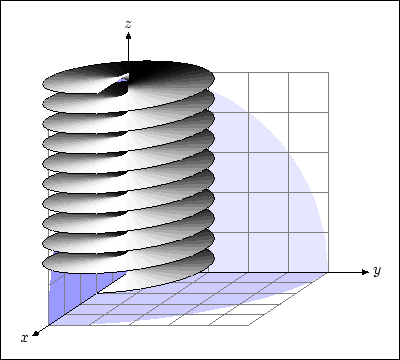In Functions.mp, the vardef...enddef construction is used to define a function «getPixel» which calculates the MetaPost coordinates of the picture from the mathematical 3D coordinates of the point to be projected.

Pxy is the projection of the 3D plane z=0 onto the mathematical 2D coordinates. Thanks to MetaPost's equation-solving capabilities, it has not to be found by sophisticated geometrical considerations; it can just be defined by it's effect on the three points (1,0), (0,1), and (0,0).

In this example, the MetaPost type color is «abused» to represent 3D vectors. Indeed, a color object is, apart from its possible use as an argument to the withcolor command, just a triplet of numbers. It can be multiplied with and divided by a numeric, and two color objects can be added.

However, it must by pointed out that for color, unlike for pair, there are no operators like unitvector or abs, and there is no transform. If such operators are needed, they must be written by the user.

In Functions.mp, the transform Txy maps the x-y plane of 3D space to the MetaPost coordinates. The z coordinate is dealt with by the getPixel subroutine.

ForSuffixes.mp

• forsuffixes
• hide
The control structure forsuffixes ... endfor allows to write «def» and «vardef» subroutines with arbitrarily varying numbers of arguments. In this example, «forsuffixes» loops are used in the subroutines «Zyklus», «Pfad», and «Lote».

By the way, the type color is the only MetaPost type which is not a type in METAFONT. So MetaPost has 9 data types, whereas METAFONT has only 8.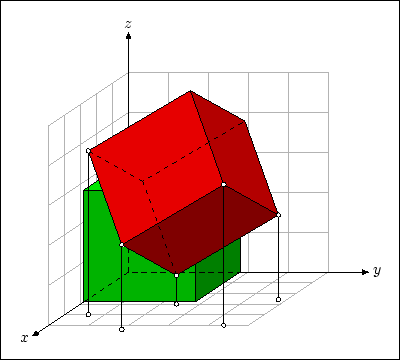For the projection of 3D points onto the MetaPost picture, the transformations t and Txy of «Functions» are used.

Notice the use of the hide command within the forsuffixes loop of the vardef subroutine Pfad(text t)!

Recursiveness.mp

Can MetaPost functions be programmed recursively?

They can. The Hilbert curves of depth 3 and 4, respectively, in the picture to the right are drawn by a recursive vardef function,

hilbert(s, n),

which returns a picture.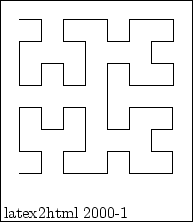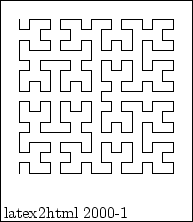For the Hilbert curve of depth 5, MetaPost capacity was exceeded [main memory size=1000001].

If you want to know more about Hilbert curves, look for "hilbert curve" with http://www.google.com or take, e.g.,
http://www.fractalus.com/kerry/tutorials/hilbert/hilbert-tutorial.html

For experts: A Hilbert curve of minimum depth zero is just a point. I made use of this fact in the recursive vardef function, hilbert(s, n). (See Recursiveness.mp .)

RecursivePath.mp

This Hilbert curve of depth 6 (and 4096 knots) was calculated recursively by the vardef subroutine

hilbertPath(s, n).

This subroutine returns a path, not a picture. The maximum depth which I could reach with main memory size=1000001 was 7 (a curve with 16384 knots). To calculate curve n from curve n-1, the following path operators are used:
• point numeric of path
• path catenation (&)
• reverse (reverse path parametrization)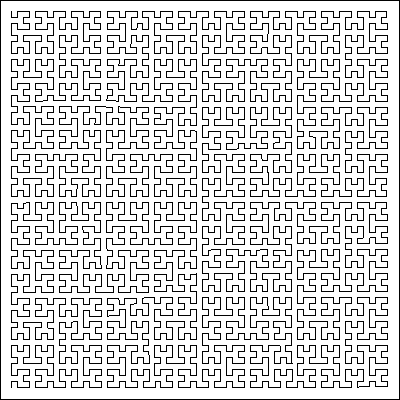ifthenInLabel.mp

The LaTeX counter i is set to 0 and stepped up to 16 in the first label. It is further stepped up to 20 in the second label. (In both cases, the final value is not shown.)

The LaTeX counter j is set to 0 and stepped up to 8 in 8 consecutive labels (red circles). However, the counter j is not stepped up if the command \stepcounter{j}' is contained in a MetaPost loop (green circles).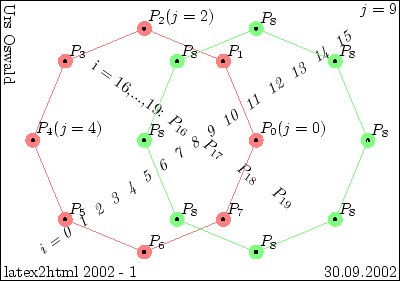NewOperators

Binary operators defined with tertiarydef, secondarydef, and primarydef expect the right argument to be of tertiary, secondary, and primary precedence level, respectively, whereas the left argument is expected to be one level off. But see the primarydef examples! /

osurs@linux:~/latex/metapost> mpost
This is MetaPost, Version 0.641 (Web2C 7.3.7)
**\relax


 *tertiarydef p terdef q = 2p+3q enddef; *show 6 terdef 35; >> 117 *show 6 terdef 4*8+3; >> 117 *show 2+2*2 terdef 35; >> 117 *show 6 terdef 4*8+3=117; >> true *show 6 terdef 4*8+3=118; >> false *show 6=2+2*2 terdef 35; >> 2 >> true ! Not implemented: (known numeric)*(boolean).  As this example shows, a binary operator terdef defined with tertiarydef expects a tertiary right argument, whereas the left argument can be of expression type. *secondarydef p secdef q = 2p+3q enddef; *show 6 secdef 35; >> 117 *show 2*3 secdef 5*7; >> 117 *show 6 secdef 4*8+3; >> 111 *show (6 secdef 4*8)+3; >> 111 *show 2+2*2 secdef 35; >> 117 *show 7=2+2*2 secdef 35; >> false  As this example shows, a binary operator secdef defined with secondarydef expects a secondary right argument, whereas the left argument can be of tertiary type. *primarydef p primdef q = 2p+3q enddef; *show 6 primdef 35; >> 117 *show 6 primdef 5*7; >> 117 *show 6 primdef 4*8+3; >> 111 *show (6 primdef 4*8)+3; >> 111  This binary operator primdef defined with primarydef also expects a secondary right argument! *primarydef p primdef\_k q = (2p+3q) enddef; *show 6 primdef\_k 5*7; >> 189 *show (6 primdef\_k 5)*7; >> 189 *show 2*3 primdef\_k 35; >> 117 *show 100+2*3 primdef\_k 35; >> 217 *show 100+(2*3 primdef\_k 35); >> 217 *end  After enclosing the value to be returned in parentheses (or BEGINGROUP ...ENDGROUP) this binary operator primdef_k defined with primarydef expects a primary right argument, whereas the left argument can be of secondary type.

DynamicTeXLabels.mp

• write ... to ...
• input ...
While MetaPost instances of type string can be manipulated arbitrarily and put into labels, this is not possible with the btex ... etex command in a direct way. It would, of course, be possible to get the figure to the right by writing a separate label command for each of the 32 vertices.

However, any MetaPost string containing an executable command can be brought to life by first writing the string to a file, and subsequently inputting this file. The input' command of MetaPost not only reads the (first line of) the file, but also automatically executes it.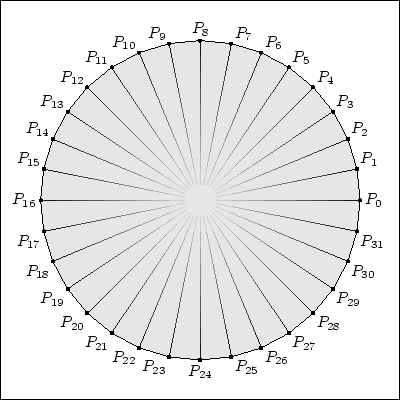To my knowledge, this possibility of writing fully dynamical TeX labels is documented neither in John D. Hobby's "User's Manual" nor in the "LaTeX Graphics Companion" of Goossens, Rahtz, and Mittelbach. I found it in the book of Alan Hoenig, TeX UNBOUND, in subsection 13.6.7.

TextOnCurve.mp

• write ... to ...
• input ...
This is another example for writing command strings to a file and subsequently execution the commands by inputting the (first line of) the saved file.

There are some restrictions to file writing and file reading commands. They cannot, i.g., be applied within macros or loops.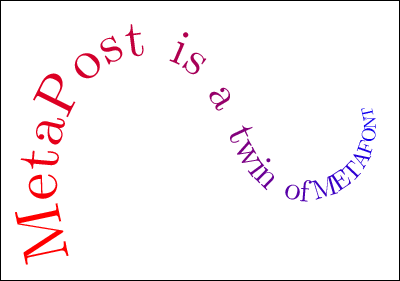As can be seen from the figure, there remain some problems to be solved. The starting points of the letters are calculated from the width of their bboxes (including the effect of scaling). bboxmargin is set to zero. However, the curvature is not taken into consideration at all. Therefore, the letters are rather too far apart when they are headed to the outside of the curve, and rather too close when they are headed to the inside.

 A2B LineSegments Circles CircleParametrization BezierCurves GraphSqrt Paths StringLabels TeXLabels LaTeXLabels Equations Mediation Precedence whatever Directions Times Colors Slanted Scaled xScaled zScaled Transform Functions ForSuffixes Recursiveness RecursivePath ifthenInLabel NewOperators DynamicTeXLabels TextOnCurve